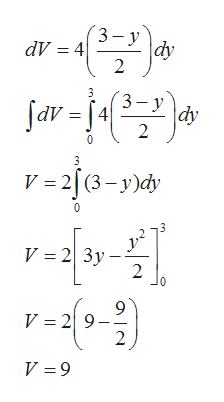# Find the volume V of the described solid S.The base of S is the region enclosed by the parabola y = 3 − 2x2 and the x−axis. Cross-sections perpendicular to the y−axis are squares. V=?

Question
474 views

Find the volume V of the described solid S.

The base of S is the region enclosed by the parabola
y = 3 − 2x2
and the x−axis. Cross-sections perpendicular to the y−axis are squares.

V=?
check_circle

Step 1

Given base of the solid S enclosed by parabola y=3-2x2 and the x axis and cross-sections are perpendicular to the y axis are squares. So first find the cross-sectional area of solid and then integrate it along y axis to find the volume.

Step 2

Cross-sectional area is in the form of Squares with side 2x and width dx is given by

Step 3

For limit of integration along y axis, put x=0 we get y=3 and also y is bounded below by ...help_outlineImage Transcriptionclosedy dv 43-y 2 3 3- y 4 sav dy 2 V 2 (3-y)dy V 2 3y 2 l0 V 29 2 V 9 fullscreen

### Want to see the full answer?

See Solution

#### Want to see this answer and more?

Solutions are written by subject experts who are available 24/7. Questions are typically answered within 1 hour.*

See Solution
*Response times may vary by subject and question.
Tagged in

### Other